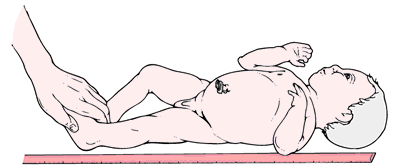# length

(redirected from cycle length)
Also found in: Dictionary, Thesaurus, Encyclopedia.

## length

[length]
an expression of the longest dimension of an object, or of the measurement between its two ends.
crown-heel length (CHL) the distance from the crown of the head to the heel in embryos, fetuses, and infants; the equivalent of standing height in older persons.Measuring an infant's crown-heel length. From Lammon et al., 1996.
crown-rump length (CRL) the distance from the crown of the head to the breech in embryos, fetuses, and infants; the equivalent of sitting height in older subjects.
cycle length in cardiac pacing terminology, the time interval in milliseconds from one event to the next; it is the inverse of the intrinsic rate (beats per minute) or the paced rate (pulses per minute).
focal length the distance between a lens and an object from which all rays of light are brought to a focus.

## length (l),

(length), Avoid the mispronunciation lenth.
Linear distance between two points.

## length

The linear distance between 2 points. See Collateral length, Pulse length, Stride length.

## length

(length)
Linear distance between two points.

## length

(length) Avoid the mispronunciation lenth.
Linear distance between two points.
References in periodicals archive ?
Dysmenorrhea was the most prevalent 140 (70 %) menstrual disorder in our sample, followed by PMS 64 (32 %), and abnormal cycle lengths 54 (27.5%)
(A-C) Average [Ca.sup.2+] alternans magnitude is plotted against the pacing cycle length (PCL) following (A) 4(n = 4/group), (B) 8- (FA n = 4; SHS n = 5), and (C) 12- (FA n = 4; SHS n = 4-5) wk exposure.
The major findings of the present investigation are as follows: 1) As the incline increased, cycle velocity, cycle length and pole swing time were reduced, while poling time and external power output rose.
For both cases, the cycle length is m = 25, and the error tolerance is [10.sup.-6].
Electrophysiology study; Surface and intracavitary ECG during WCT showing a cycle length of 286 ms
Comparison of the original data set with the replacement cycles PARAMETER UNIT MEASUREMENT REPLACEMENT CYCLE 1 cycle length [km] 53244.76 34.19 duration [s] 4180675.00 2791.00 duration velocity >0 [s] 3747041.00 2497.00 portion of velocity.
Fig.s in (a) show the average throughput, Fig.s in (b) show the average delay, and Fig.s in (c) show the total energy consumption under varying data arrival rate, cycle length, and number of nodes in the network.
Through the reactivity analysis at the EOC, the [U.sub.3][Si.sub.2]-FeCrAl fuel-cladding combination is suggested in the following section to replace the current combination in the condition of no reduction in the cycle length.
Dividing the total cost by the average cycle length (Q+ES)/[[mu].sub.D], which takes into account the length of shortage period, gives the average total cost per period for the four cases in (7).
Suppose the cycle length is T; the discretization is done as
Baseline total cycle length (TCL) was 280 ms [Figure 1]a.
Differences in cycle length may have impacted the results of this study.

Site: Follow: Share:
Open / Close